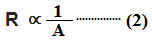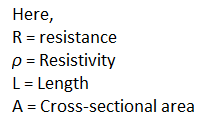## Resistance Formula

Resistance is a very well known and important word in electrical engineering as well as electronics engineering. In any electrical calculation, the resistance must include. Today we are going to know Resistance Formula.

Resistance: The property of a resistor by virtue of which the resistor can oppose the flow of electric current(Both AC and DC) is called Resistance.

In the case of wire, if the length of the wire is more then the resistance will more and if the length is less the resistance will also less.The resistance will be increased if the cross-sectional area of the wire is decreased and the resistance will be decreased if the cross-sectional area of the wire is increased.From (1) and (2) we can write,The unit of resistance is Ohm which is denoted by Ω

According to the Ohm's law if the temperature and other physical quantity is constant then the resistance formula is,Here,
R = resistance
V = Voltage across the Resistor
I = Current flowing through the resistor

So the formula of the voltage drop across the resistor is,

V = IR

The formula of Power loss across the resistor is,Resistance heating effect equation,### Resistors in series:

If a no. of resistors are connected in series then the formula of resistance will be,### Resistors in Parallel:

If a no. of resistors are connected in parallel then the formula of resistance will be,### Let's Understand Resistance Formula with Examples:

Q.1. An electrical circuit has two 5 ohm resistors, one 10 ohm resistor,  and five 2 ohm resistors in series what is the total resistance of the Circuit?

Ans: The total resistance will be,Q.2. In an electrical circuit, a 15 ohm resistor is connected with 20 ohm resistor in parallel what is the total resistance of the circuit?

Ans: The total effective resistance will be,Q.3. An electrical circuit has 5 ohm, 3 ohm, 4 ohm resistors in series. If the circuit is connected to the 12V DC supply what is voltage drop across the 3 ohm resistor?

Ans: At first we need to find the total resistance of the circuit. As all resistors are connected in series so the total resistance will be,

R = (5+3+4)
= 12 ohm

So the current flowing through the circuit is, I = V/R
= 12/12
= 1 amp

So the voltage drop across the 3 ohm resistor will be,
V=IR
= 1 × 3
= 3 volt

Resistance Formula | All Formulas and Equations ExplainReviewed by manoranjan das on 9:47 am Rating: 5